Скачать презентацию Electronic Filing and Calculating History of Calculators

15d60808b78eaeaf489ed2b7311c700d.ppt

• Количество слайдов: 58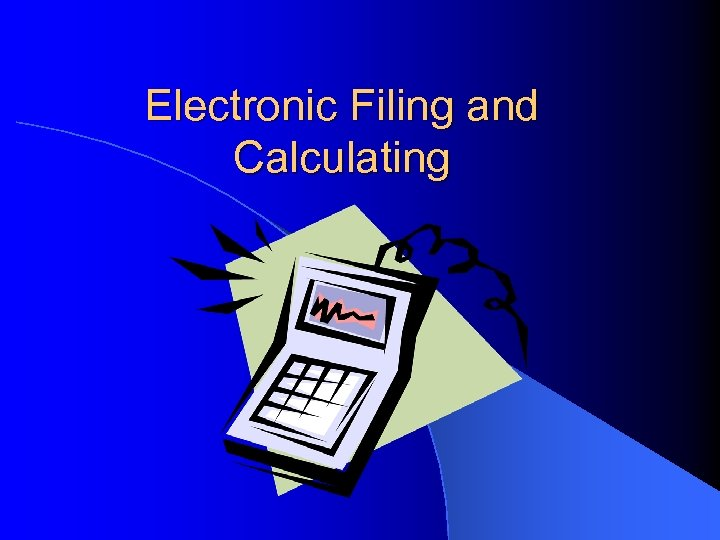Electronic Filing and Calculating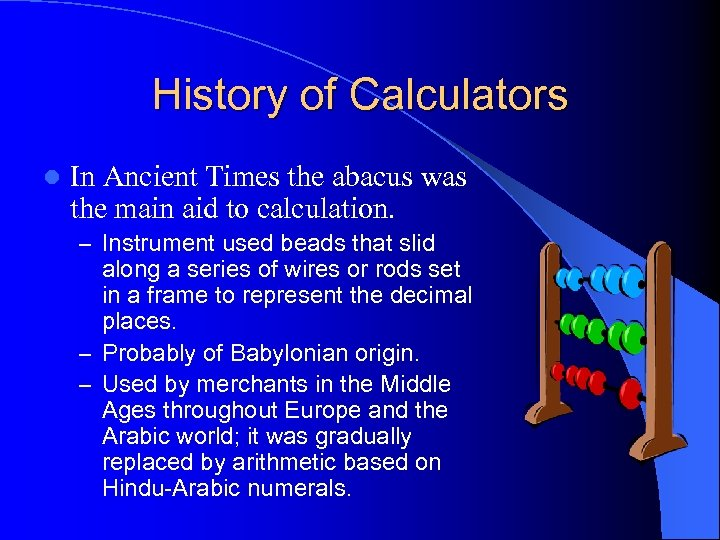History of Calculators l In Ancient Times the abacus was the main aid to calculation. – Instrument used beads that slid along a series of wires or rods set in a frame to represent the decimal places. – Probably of Babylonian origin. – Used by merchants in the Middle Ages throughout Europe and the Arabic world; it was gradually replaced by arithmetic based on Hindu-Arabic numerals.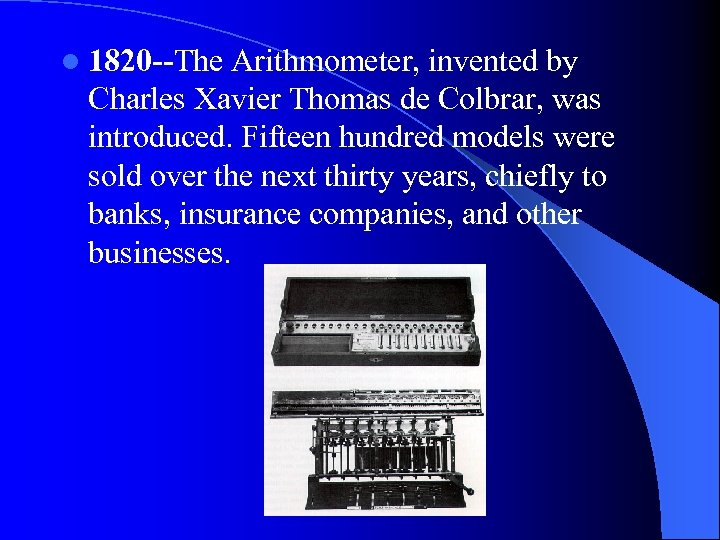l 1820 --The Arithmometer, invented by Charles Xavier Thomas de Colbrar, was introduced. Fifteen hundred models were sold over the next thirty years, chiefly to banks, insurance companies, and other businesses.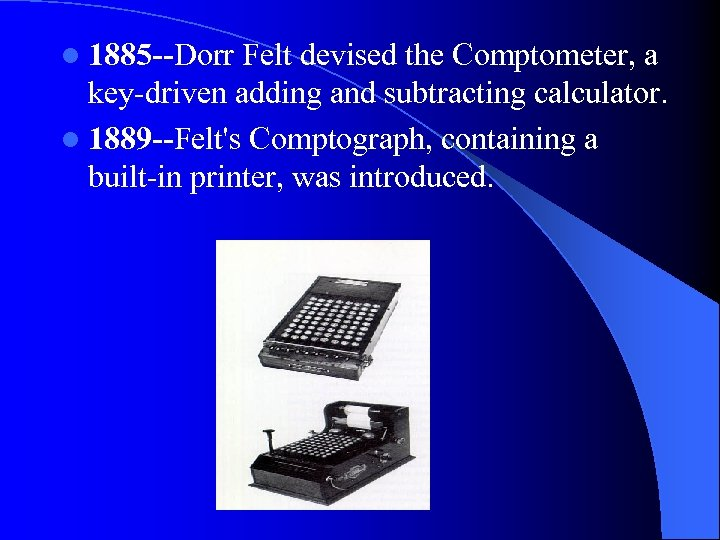l 1885 --Dorr Felt devised the Comptometer, a key-driven adding and subtracting calculator. l 1889 --Felt's Comptograph, containing a built-in printer, was introduced.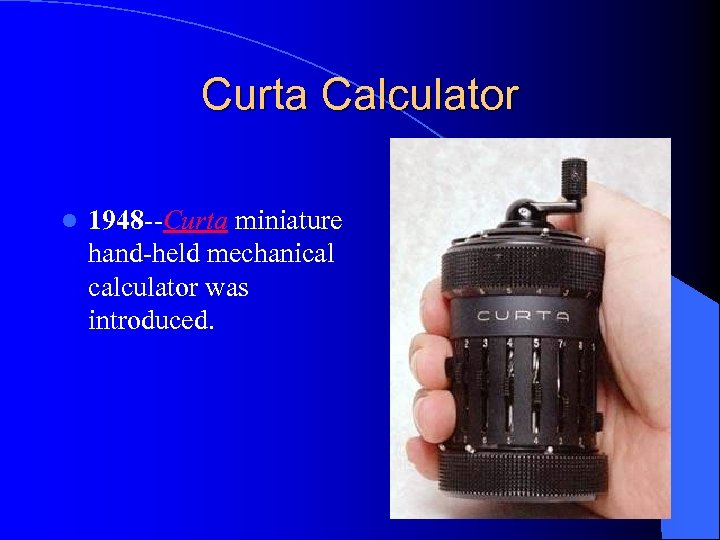Curta Calculator l 1948 --Curta miniature hand-held mechanical calculator was introduced.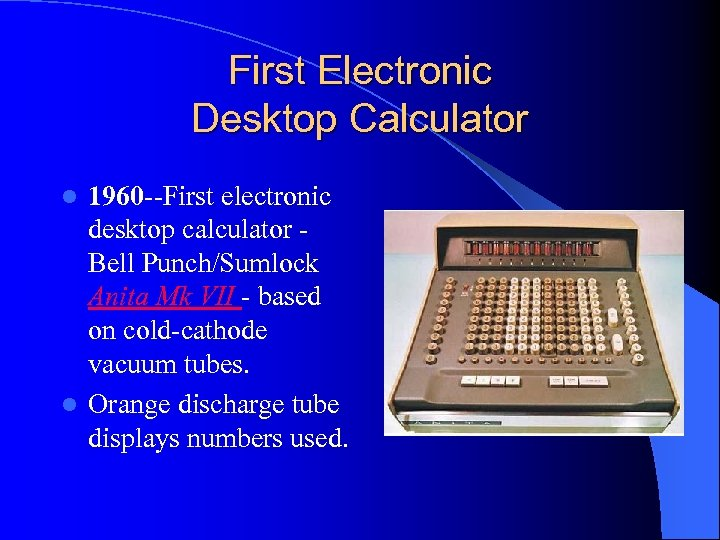First Electronic Desktop Calculator 1960 --First electronic desktop calculator Bell Punch/Sumlock Anita Mk VII - based on cold-cathode vacuum tubes. l Orange discharge tube displays numbers used. l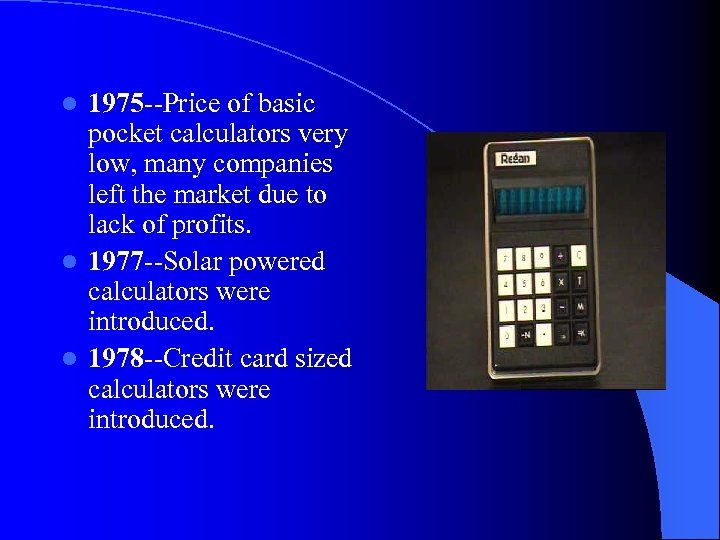1975 --Price of basic pocket calculators very low, many companies left the market due to lack of profits. l 1977 --Solar powered calculators were introduced. l 1978 --Credit card sized calculators were introduced. l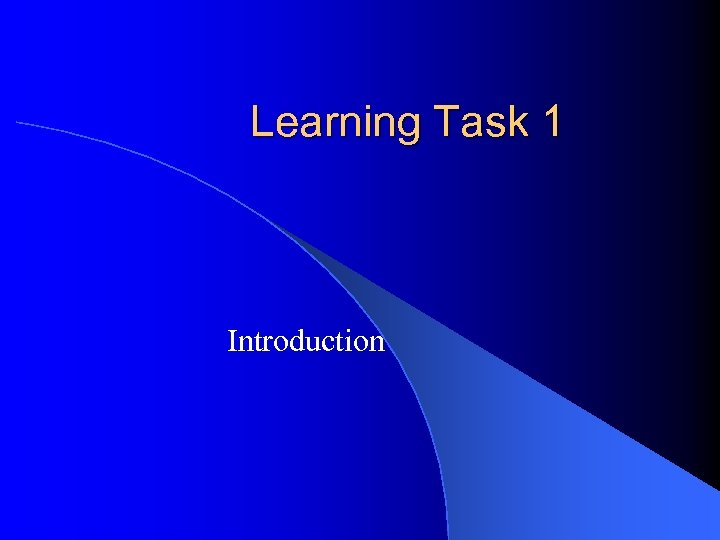Learning Task 1 Introduction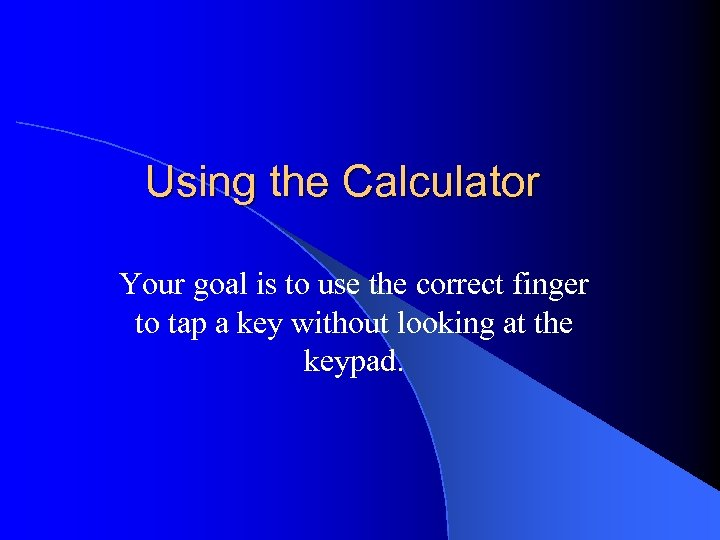Using the Calculator Your goal is to use the correct finger to tap a key without looking at the keypad.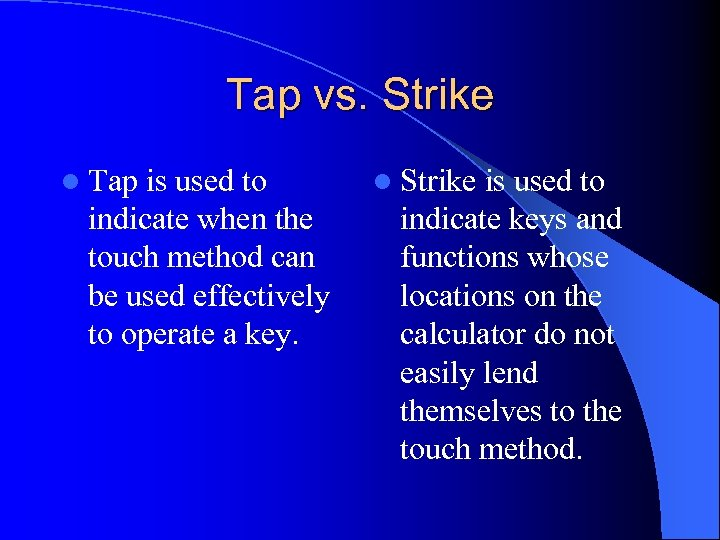Tap vs. Strike l Tap is used to indicate when the touch method can be used effectively to operate a key. l Strike is used to indicate keys and functions whose locations on the calculator do not easily lend themselves to the touch method.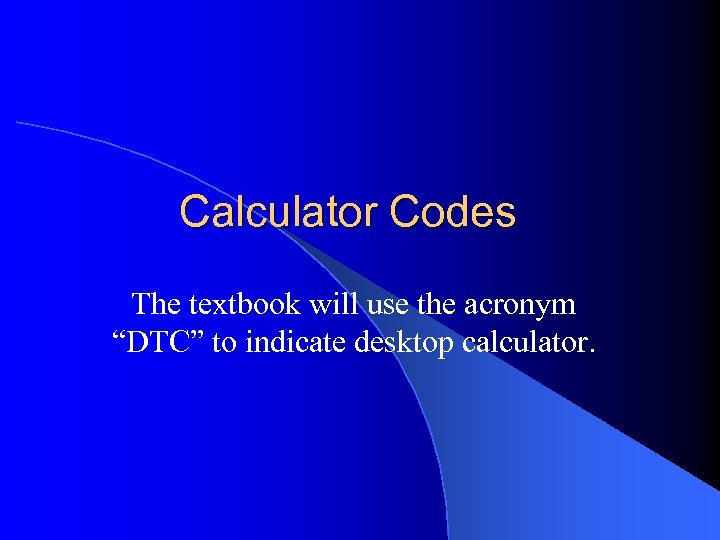Calculator Codes The textbook will use the acronym “DTC” to indicate desktop calculator.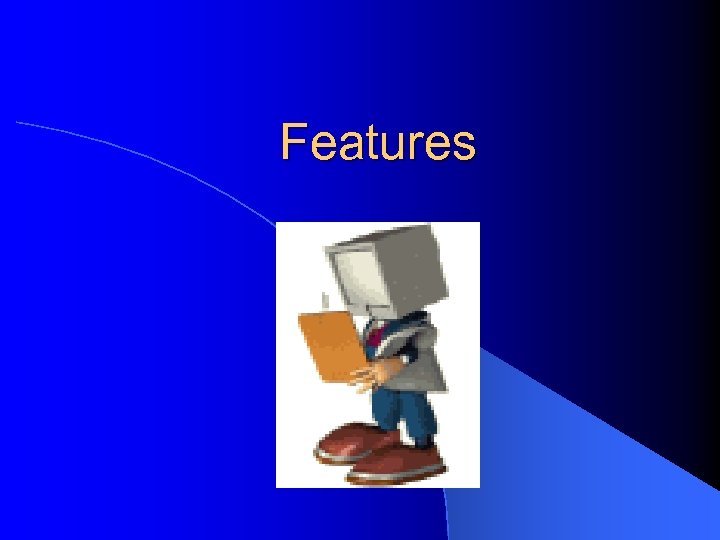Features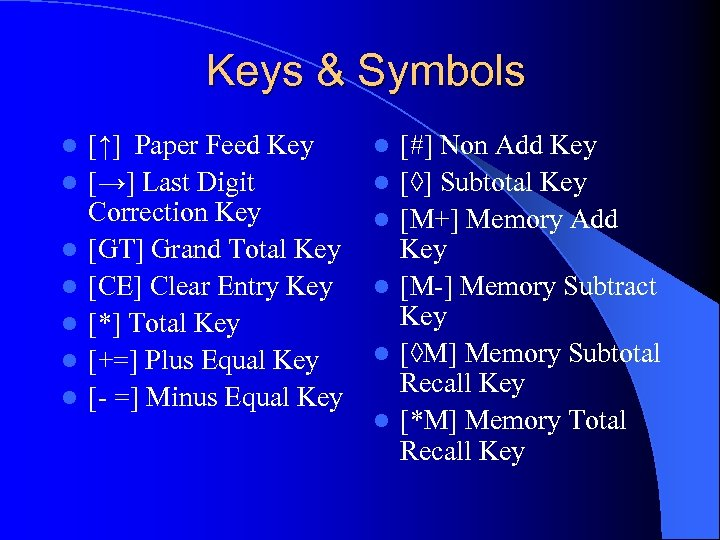Keys & Symbols l l l l [↑] Paper Feed Key [→] Last Digit Correction Key [GT] Grand Total Key [CE] Clear Entry Key [*] Total Key [+=] Plus Equal Key [- =] Minus Equal Key l l l [#] Non Add Key [◊] Subtotal Key [M+] Memory Add Key [M-] Memory Subtract Key [◊M] Memory Subtotal Recall Key [*M] Memory Total Recall Key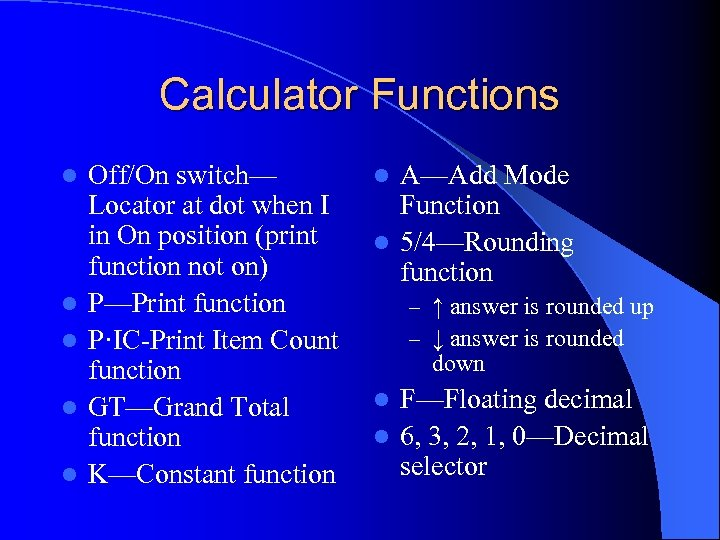Calculator Functions l l l Off/On switch— Locator at dot when I in On position (print function not on) P—Print function P·IC-Print Item Count function GT—Grand Total function K—Constant function A—Add Mode Function l 5/4—Rounding function l – ↑ answer is rounded up – ↓ answer is rounded down F—Floating decimal l 6, 3, 2, 1, 0—Decimal selector l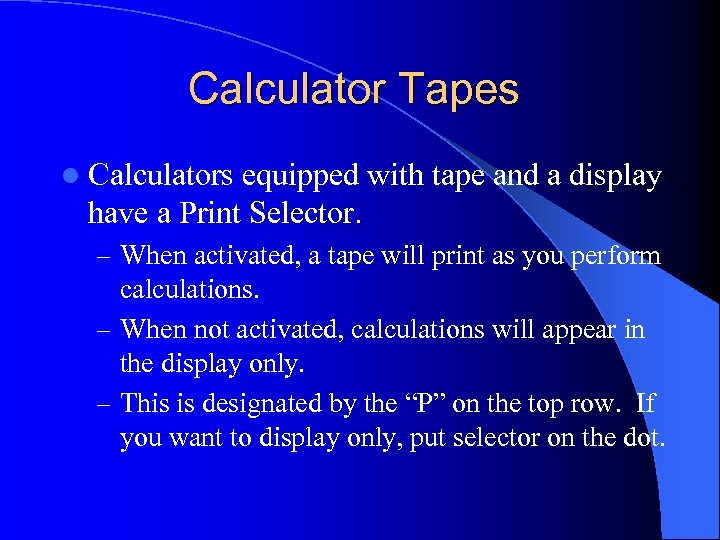Calculator Tapes l Calculators equipped with tape and a display have a Print Selector. – When activated, a tape will print as you perform calculations. – When not activated, calculations will appear in the display only. – This is designated by the “P” on the top row. If you want to display only, put selector on the dot.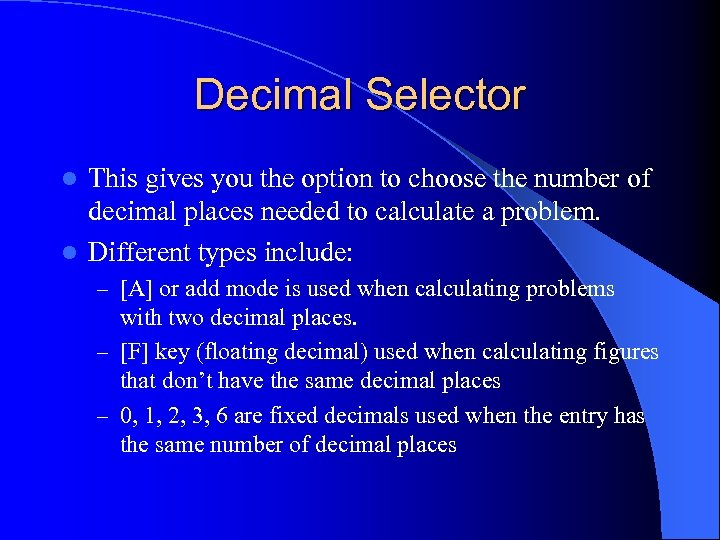Decimal Selector This gives you the option to choose the number of decimal places needed to calculate a problem. l Different types include: l – [A] or add mode is used when calculating problems with two decimal places. – [F] key (floating decimal) used when calculating figures that don’t have the same decimal places – 0, 1, 2, 3, 6 are fixed decimals used when the entry has the same number of decimal places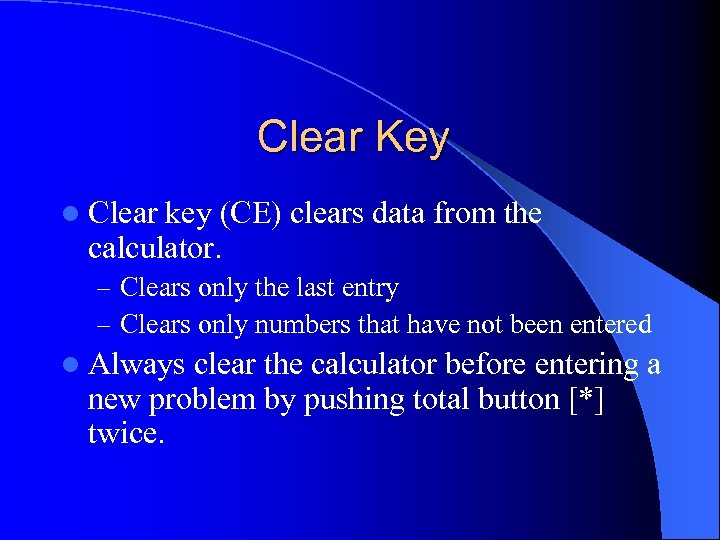Clear Key l Clear key (CE) clears data from the calculator. – Clears only the last entry – Clears only numbers that have not been entered l Always clear the calculator before entering a new problem by pushing total button [*] twice.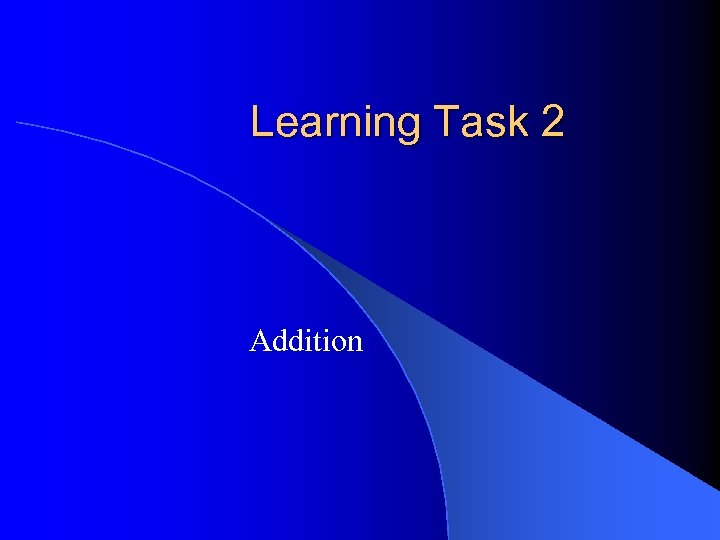Learning Task 2 Addition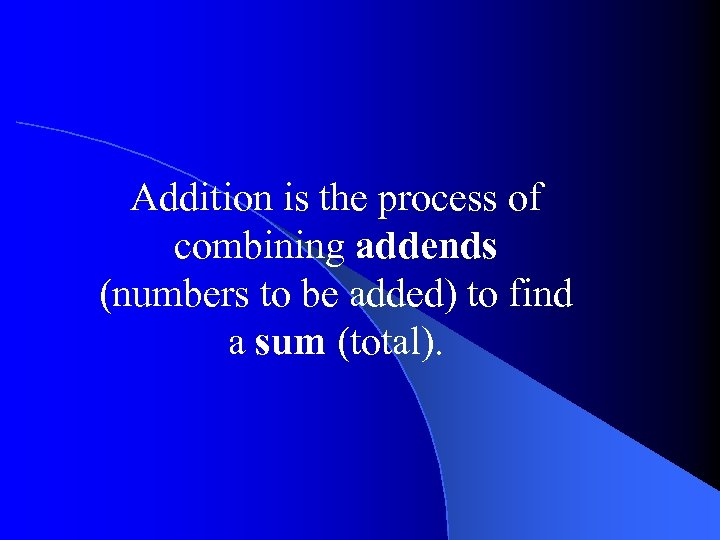Addition is the process of combining addends (numbers to be added) to find a sum (total).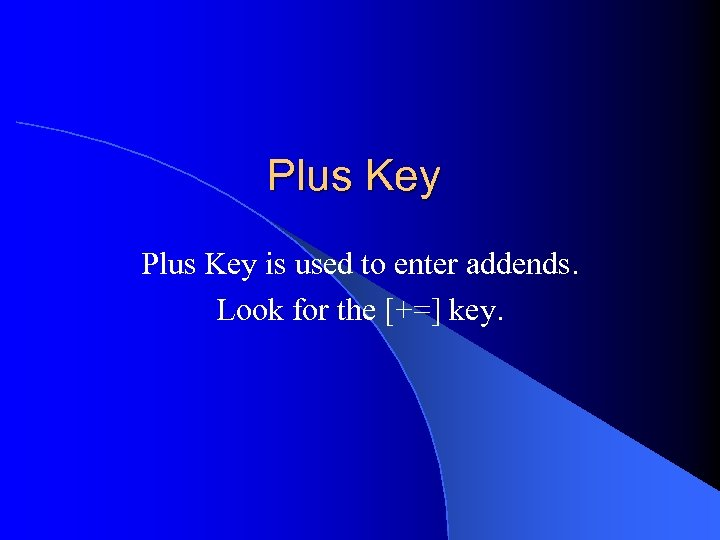Plus Key is used to enter addends. Look for the [+=] key.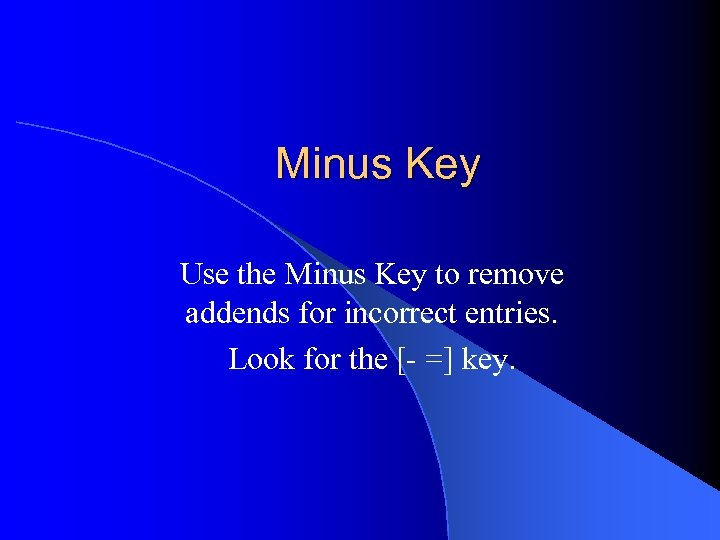Minus Key Use the Minus Key to remove addends for incorrect entries. Look for the [- =] key.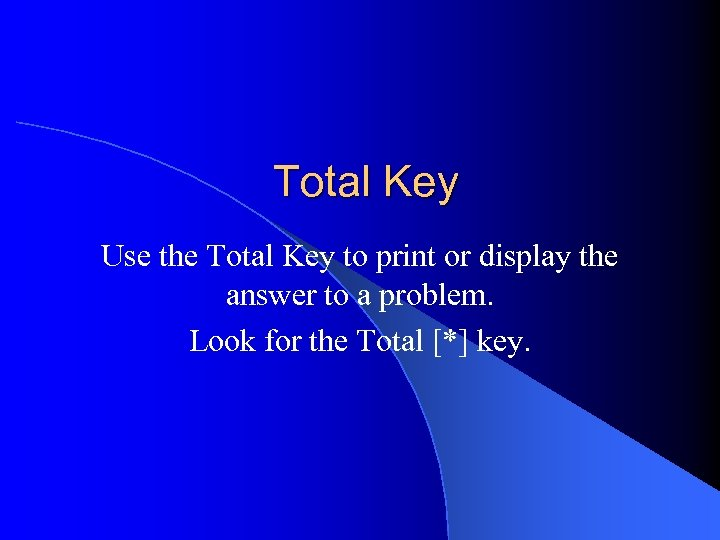Total Key Use the Total Key to print or display the answer to a problem. Look for the Total [*] key.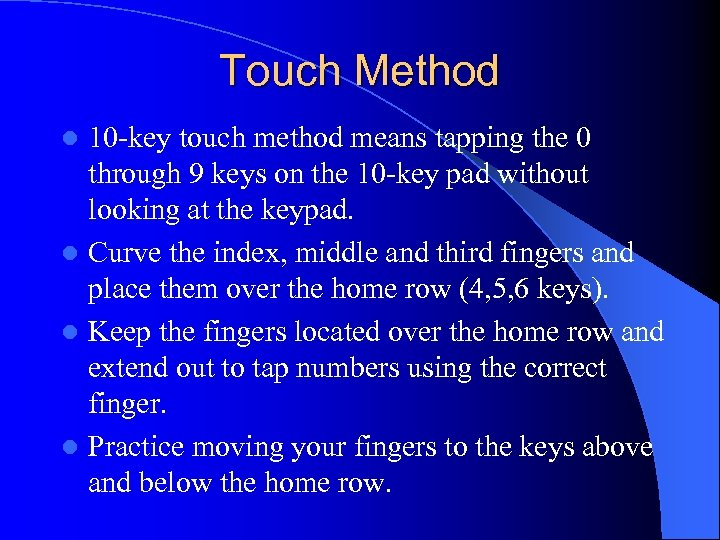Touch Method 10 -key touch method means tapping the 0 through 9 keys on the 10 -key pad without looking at the keypad. l Curve the index, middle and third fingers and place them over the home row (4, 5, 6 keys). l Keep the fingers located over the home row and extend out to tap numbers using the correct finger. l Practice moving your fingers to the keys above and below the home row. l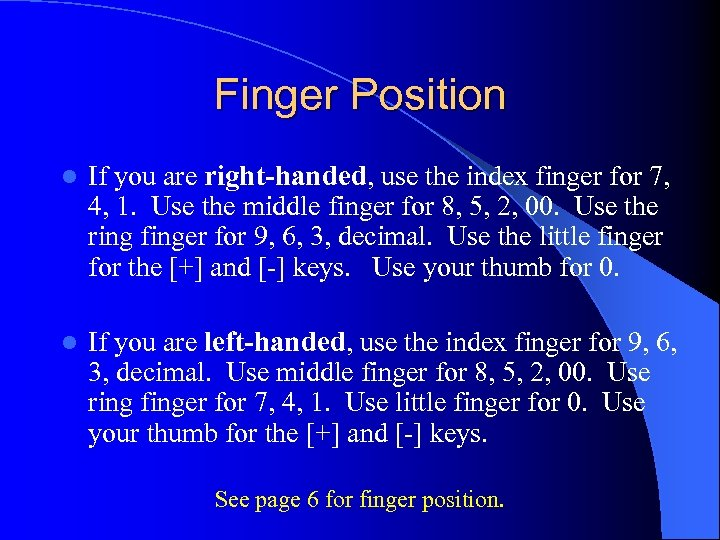Finger Position l If you are right-handed, use the index finger for 7, 4, 1. Use the middle finger for 8, 5, 2, 00. Use the ring finger for 9, 6, 3, decimal. Use the little finger for the [+] and [-] keys. Use your thumb for 0. l If you are left-handed, use the index finger for 9, 6, 3, decimal. Use middle finger for 8, 5, 2, 00. Use ring finger for 7, 4, 1. Use little finger for 0. Use your thumb for the [+] and [-] keys. See page 6 for finger position.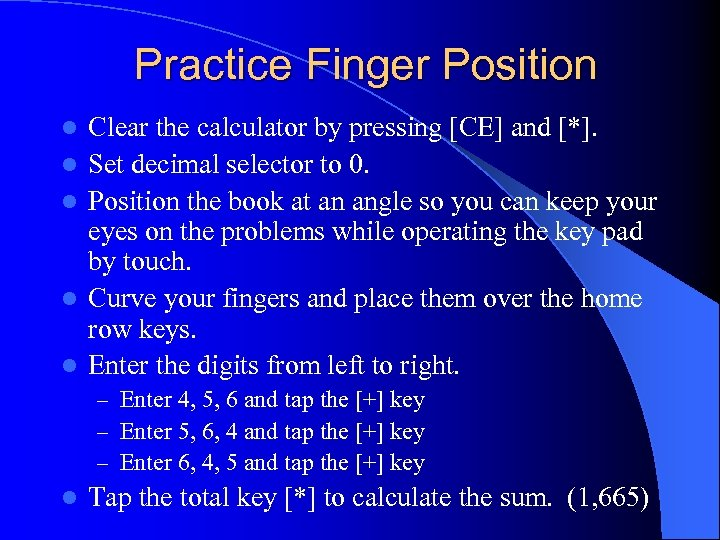Practice Finger Position l l l Clear the calculator by pressing [CE] and [*]. Set decimal selector to 0. Position the book at an angle so you can keep your eyes on the problems while operating the key pad by touch. Curve your fingers and place them over the home row keys. Enter the digits from left to right. – Enter 4, 5, 6 and tap the [+] key – Enter 5, 6, 4 and tap the [+] key – Enter 6, 4, 5 and tap the [+] key l Tap the total key [*] to calculate the sum. (1, 665)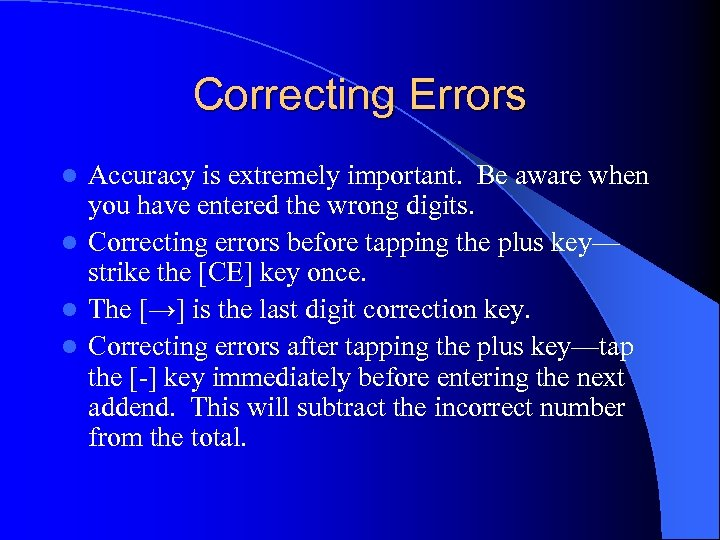Correcting Errors Accuracy is extremely important. Be aware when you have entered the wrong digits. l Correcting errors before tapping the plus key— strike the [CE] key once. l The [→] is the last digit correction key. l Correcting errors after tapping the plus key—tap the [-] key immediately before entering the next addend. This will subtract the incorrect number from the total. l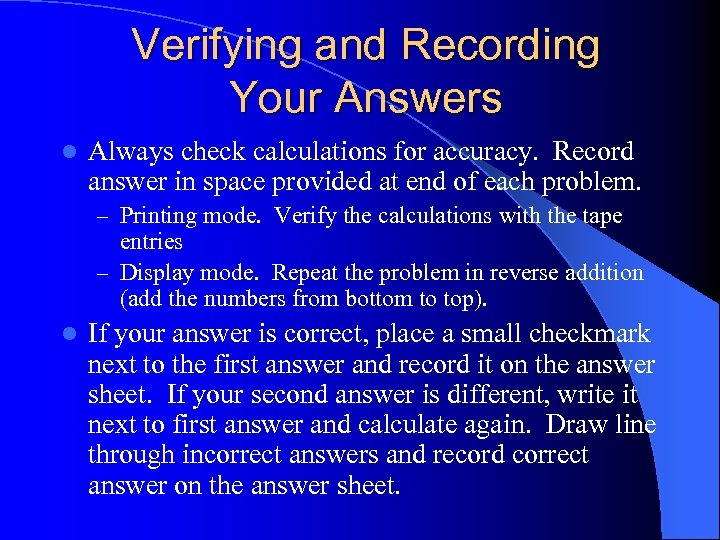Verifying and Recording Your Answers l Always check calculations for accuracy. Record answer in space provided at end of each problem. – Printing mode. Verify the calculations with the tape entries – Display mode. Repeat the problem in reverse addition (add the numbers from bottom to top). l If your answer is correct, place a small checkmark next to the first answer and record it on the answer sheet. If your second answer is different, write it next to first answer and calculate again. Draw line through incorrect answers and record correct answer on the answer sheet.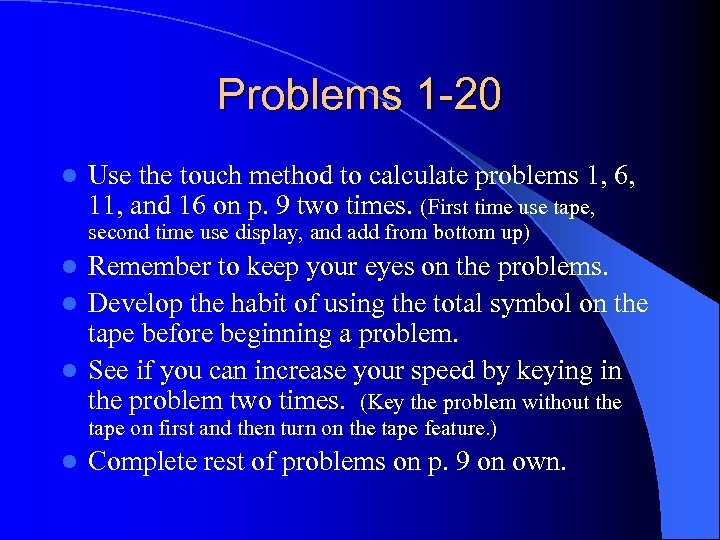Problems 1 -20 l Use the touch method to calculate problems 1, 6, 11, and 16 on p. 9 two times. (First time use tape, second time use display, and add from bottom up) Remember to keep your eyes on the problems. l Develop the habit of using the total symbol on the tape before beginning a problem. l See if you can increase your speed by keying in the problem two times. (Key the problem without the l tape on first and then turn on the tape feature. ) l Complete rest of problems on p. 9 on own.Special Features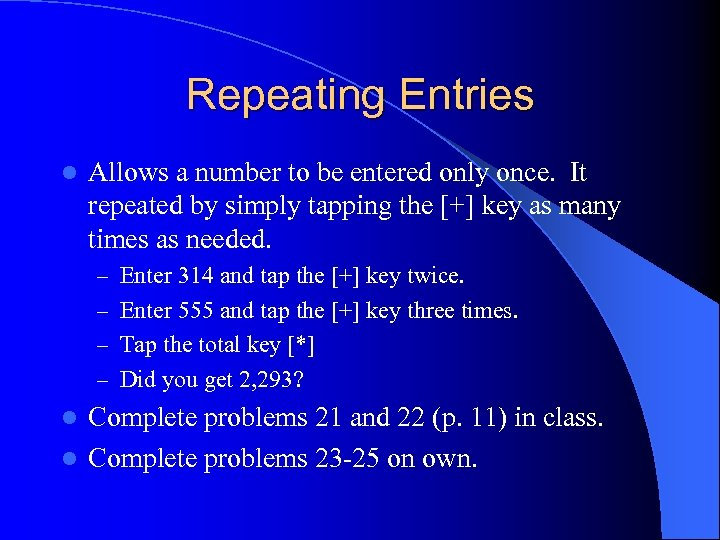Repeating Entries l Allows a number to be entered only once. It repeated by simply tapping the [+] key as many times as needed. – Enter 314 and tap the [+] key twice. – Enter 555 and tap the [+] key three times. – Tap the total key [*] – Did you get 2, 293? Complete problems 21 and 22 (p. 11) in class. l Complete problems 23 -25 on own. l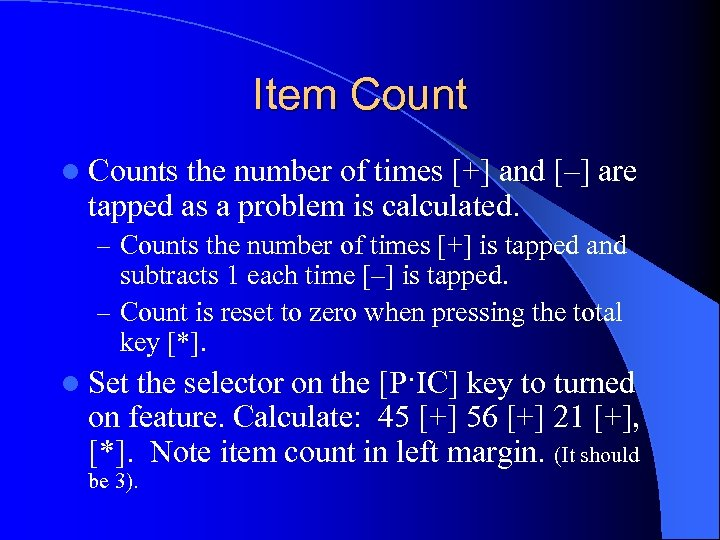Item Count l Counts the number of times [+] and [–] are tapped as a problem is calculated. – Counts the number of times [+] is tapped and subtracts 1 each time [–] is tapped. – Count is reset to zero when pressing the total key [*]. l Set the selector on the [P·IC] key to turned on feature. Calculate: 45 [+] 56 [+] 21 [+], [*]. Note item count in left margin. (It should be 3).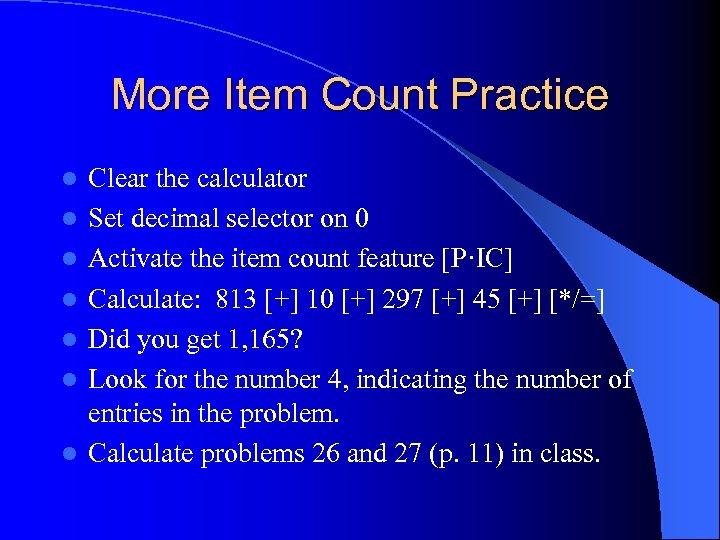More Item Count Practice l l l l Clear the calculator Set decimal selector on 0 Activate the item count feature [P·IC] Calculate: 813 [+] 10 [+] 297 [+] 45 [+] [*/=] Did you get 1, 165? Look for the number 4, indicating the number of entries in the problem. Calculate problems 26 and 27 (p. 11) in class.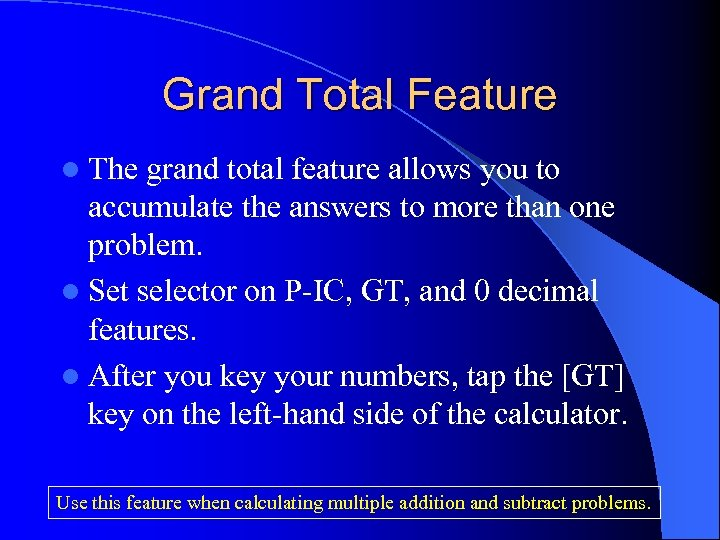Grand Total Feature l The grand total feature allows you to accumulate the answers to more than one problem. l Set selector on P-IC, GT, and 0 decimal features. l After you key your numbers, tap the [GT] key on the left-hand side of the calculator. Use this feature when calculating multiple addition and subtract problems.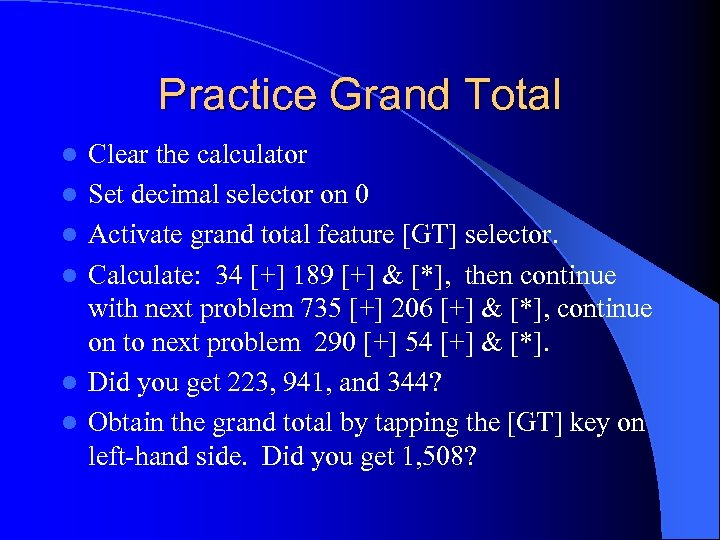Practice Grand Total l l l Clear the calculator Set decimal selector on 0 Activate grand total feature [GT] selector. Calculate: 34 [+] 189 [+] & [*], then continue with next problem 735 [+] 206 [+] & [*], continue on to next problem 290 [+] 54 [+] & [*]. Did you get 223, 941, and 344? Obtain the grand total by tapping the [GT] key on left-hand side. Did you get 1, 508?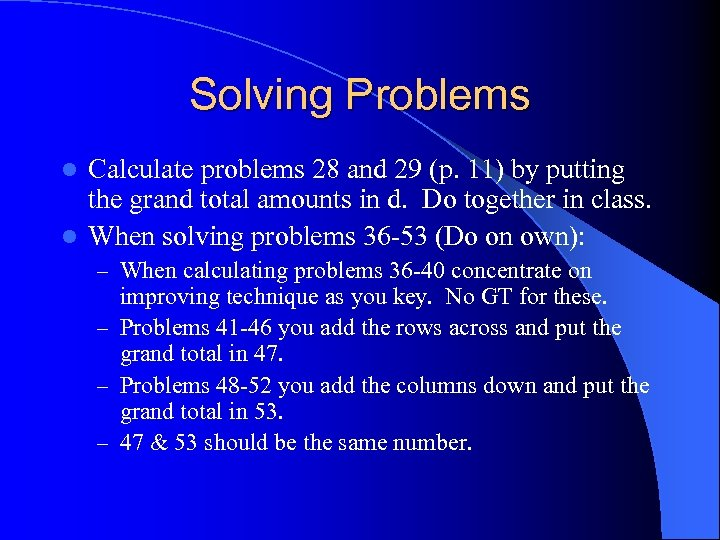Solving Problems Calculate problems 28 and 29 (p. 11) by putting the grand total amounts in d. Do together in class. l When solving problems 36 -53 (Do on own): l – When calculating problems 36 -40 concentrate on improving technique as you key. No GT for these. – Problems 41 -46 you add the rows across and put the grand total in 47. – Problems 48 -52 you add the columns down and put the grand total in 53. – 47 & 53 should be the same number.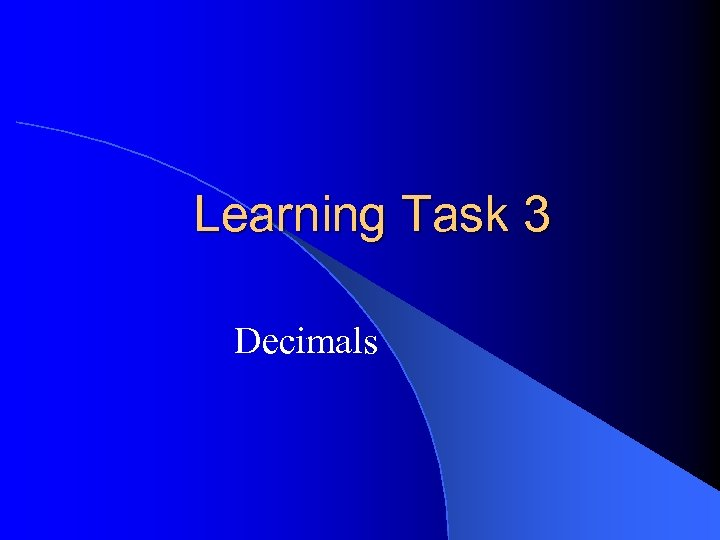Learning Task 3 Decimals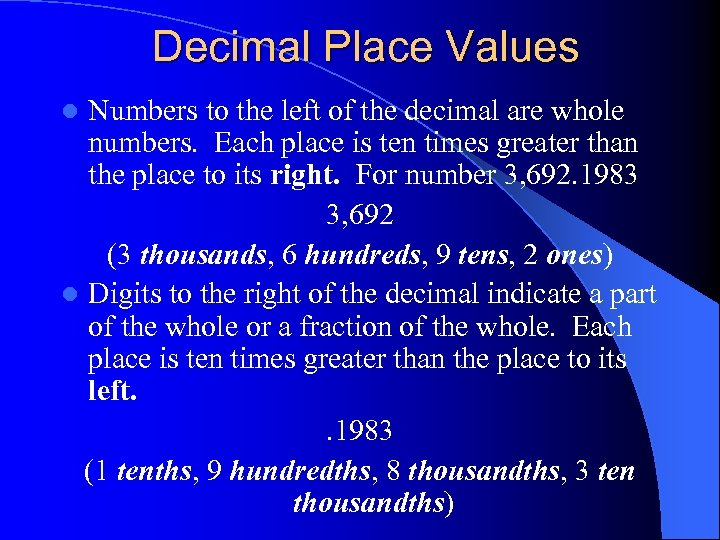Decimal Place Values Numbers to the left of the decimal are whole numbers. Each place is ten times greater than the place to its right. For number 3, 692. 1983 3, 692 (3 thousands, 6 hundreds, 9 tens, 2 ones) l Digits to the right of the decimal indicate a part of the whole or a fraction of the whole. Each place is ten times greater than the place to its left. . 1983 (1 tenths, 9 hundredths, 8 thousandths, 3 ten thousandths) l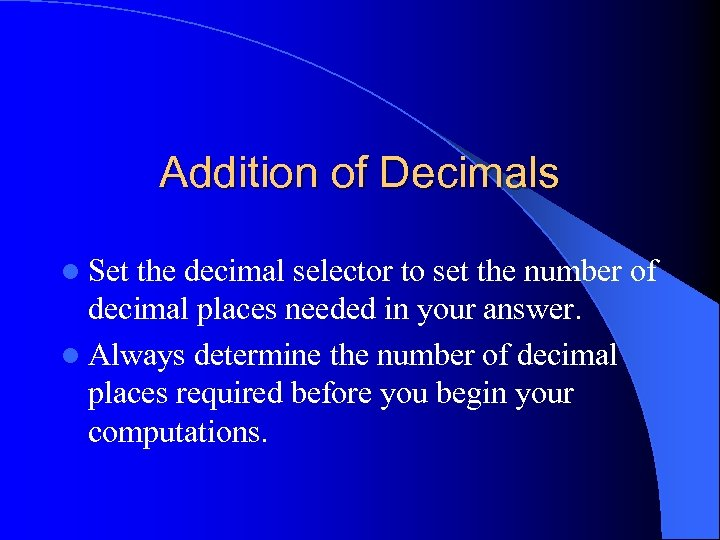Addition of Decimals l Set the decimal selector to set the number of decimal places needed in your answer. l Always determine the number of decimal places required before you begin your computations.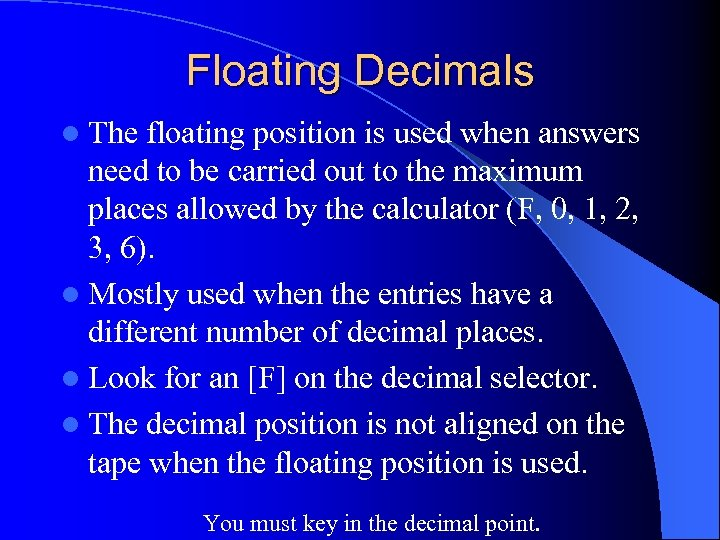Floating Decimals l The floating position is used when answers need to be carried out to the maximum places allowed by the calculator (F, 0, 1, 2, 3, 6). l Mostly used when the entries have a different number of decimal places. l Look for an [F] on the decimal selector. l The decimal position is not aligned on the tape when the floating position is used. You must key in the decimal point.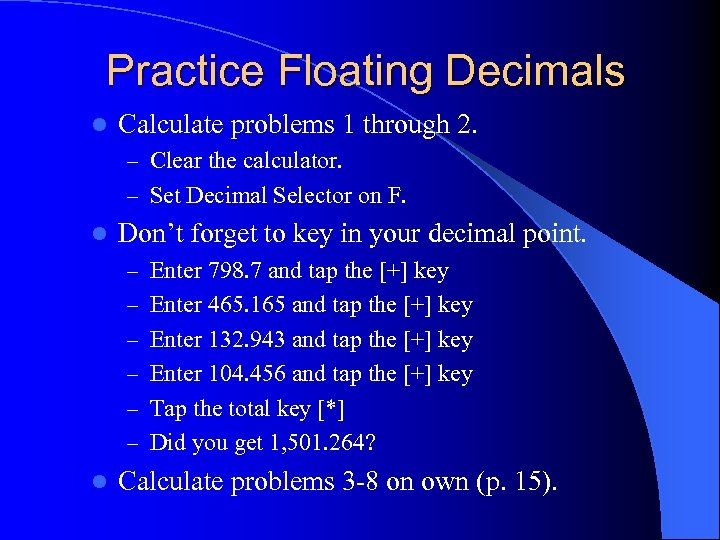Practice Floating Decimals l Calculate problems 1 through 2. – Clear the calculator. – Set Decimal Selector on F. l Don’t forget to key in your decimal point. – Enter 798. 7 and tap the [+] key – Enter 465. 165 and tap the [+] key – Enter 132. 943 and tap the [+] key – Enter 104. 456 and tap the [+] key – Tap the total key [*] – Did you get 1, 501. 264? l Calculate problems 3 -8 on own (p. 15).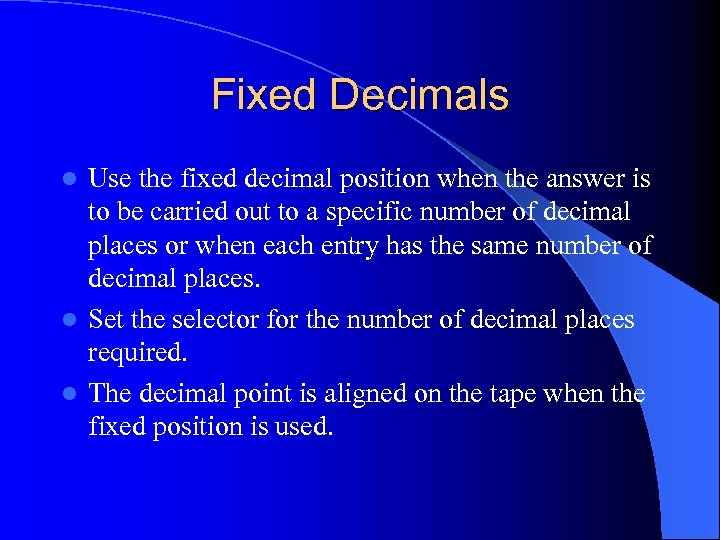Fixed Decimals Use the fixed decimal position when the answer is to be carried out to a specific number of decimal places or when each entry has the same number of decimal places. l Set the selector for the number of decimal places required. l The decimal point is aligned on the tape when the fixed position is used. l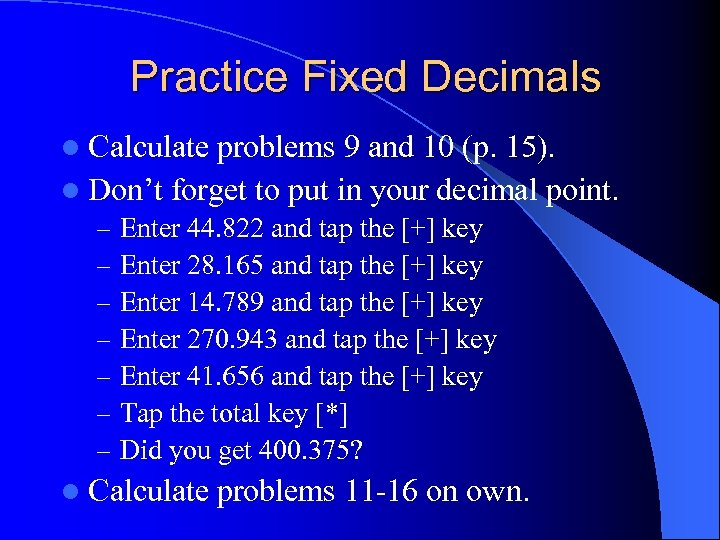Practice Fixed Decimals l Calculate problems 9 and 10 (p. 15). l Don’t forget to put in your decimal point. – – – – Enter 44. 822 and tap the [+] key Enter 28. 165 and tap the [+] key Enter 14. 789 and tap the [+] key Enter 270. 943 and tap the [+] key Enter 41. 656 and tap the [+] key Tap the total key [*] Did you get 400. 375? l Calculate problems 11 -16 on own.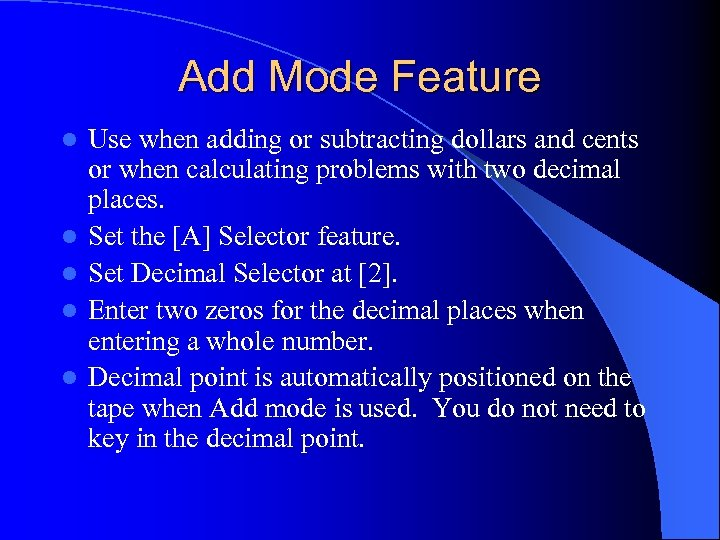Add Mode Feature l l l Use when adding or subtracting dollars and cents or when calculating problems with two decimal places. Set the [A] Selector feature. Set Decimal Selector at . Enter two zeros for the decimal places when entering a whole number. Decimal point is automatically positioned on the tape when Add mode is used. You do not need to key in the decimal point.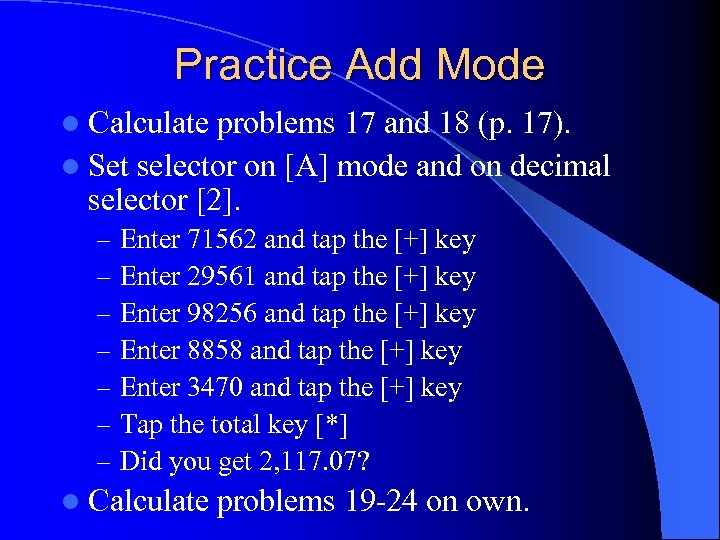Practice Add Mode l Calculate problems 17 and 18 (p. 17). l Set selector on [A] mode and on decimal selector . – – – – Enter 71562 and tap the [+] key Enter 29561 and tap the [+] key Enter 98256 and tap the [+] key Enter 8858 and tap the [+] key Enter 3470 and tap the [+] key Tap the total key [*] Did you get 2, 117. 07? l Calculate problems 19 -24 on own.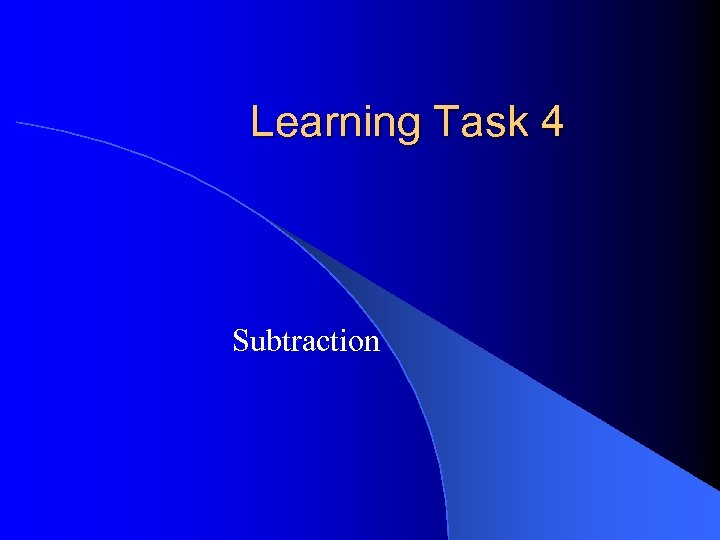Learning Task 4 Subtraction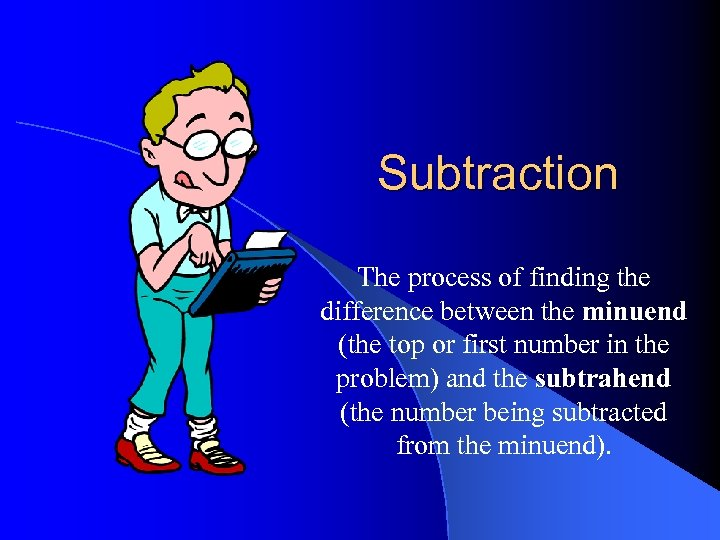Subtraction The process of finding the difference between the minuend (the top or first number in the problem) and the subtrahend (the number being subtracted from the minuend).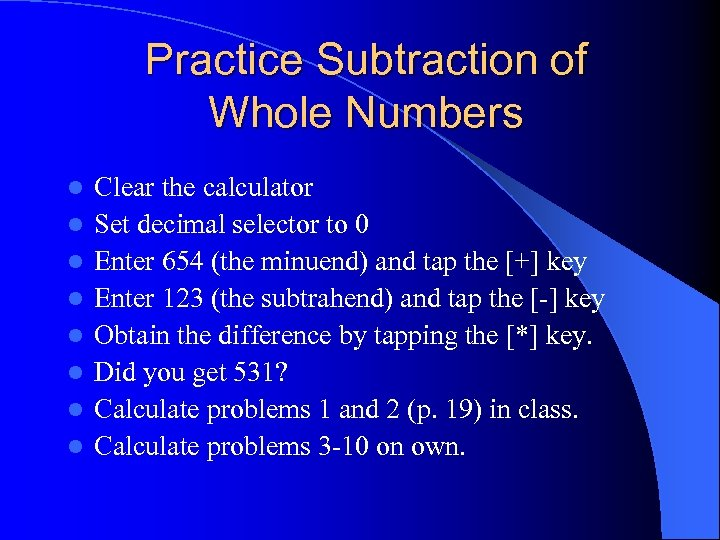Practice Subtraction of Whole Numbers l l l l Clear the calculator Set decimal selector to 0 Enter 654 (the minuend) and tap the [+] key Enter 123 (the subtrahend) and tap the [-] key Obtain the difference by tapping the [*] key. Did you get 531? Calculate problems 1 and 2 (p. 19) in class. Calculate problems 3 -10 on own.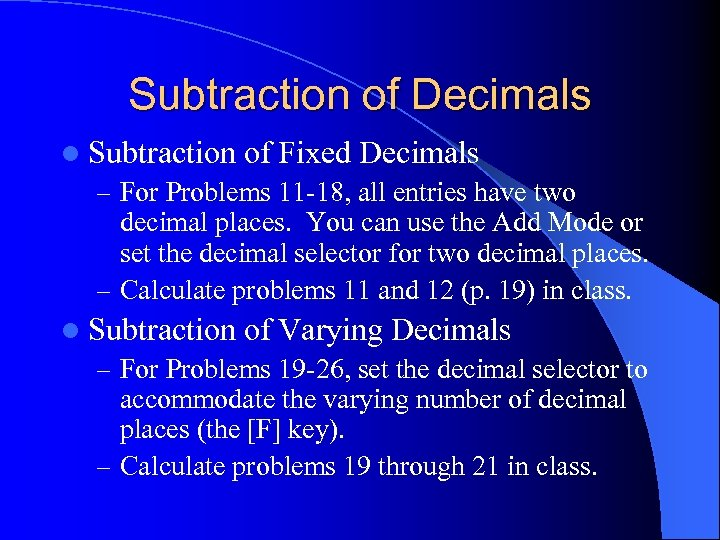Subtraction of Decimals l Subtraction of Fixed Decimals – For Problems 11 -18, all entries have two decimal places. You can use the Add Mode or set the decimal selector for two decimal places. – Calculate problems 11 and 12 (p. 19) in class. l Subtraction of Varying Decimals – For Problems 19 -26, set the decimal selector to accommodate the varying number of decimal places (the [F] key). – Calculate problems 19 through 21 in class.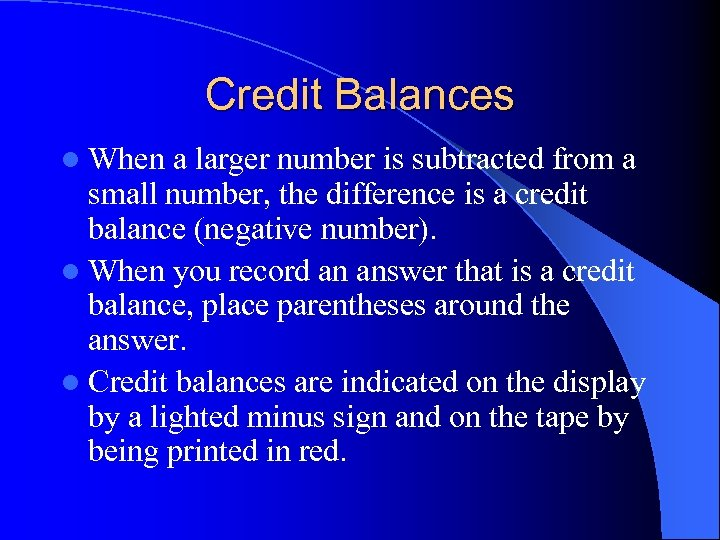Credit Balances l When a larger number is subtracted from a small number, the difference is a credit balance (negative number). l When you record an answer that is a credit balance, place parentheses around the answer. l Credit balances are indicated on the display by a lighted minus sign and on the tape by being printed in red.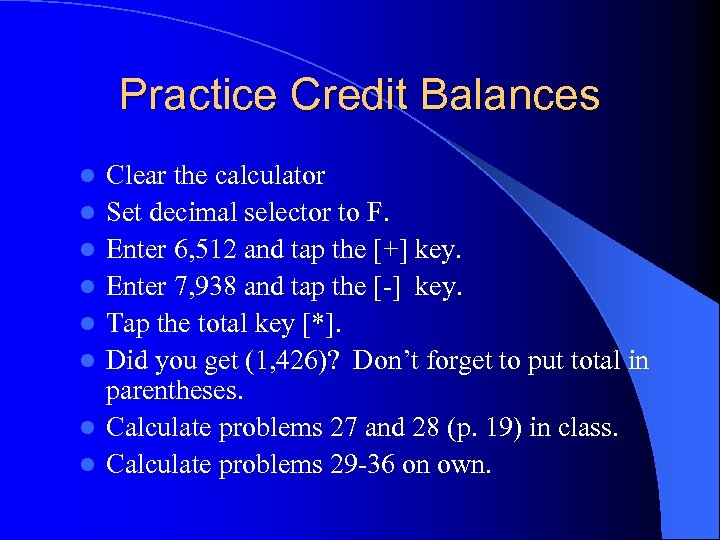Practice Credit Balances l l l l Clear the calculator Set decimal selector to F. Enter 6, 512 and tap the [+] key. Enter 7, 938 and tap the [-] key. Tap the total key [*]. Did you get (1, 426)? Don’t forget to put total in parentheses. Calculate problems 27 and 28 (p. 19) in class. Calculate problems 29 -36 on own.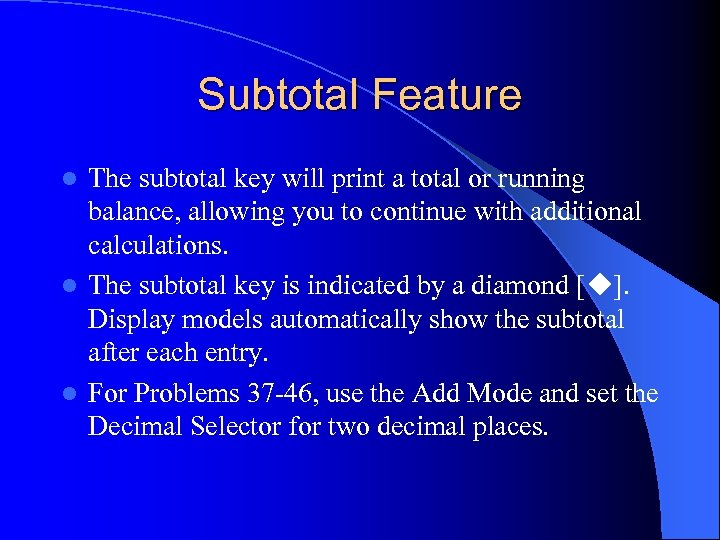Subtotal Feature The subtotal key will print a total or running balance, allowing you to continue with additional calculations. l The subtotal key is indicated by a diamond [ ]. Display models automatically show the subtotal after each entry. l For Problems 37 -46, use the Add Mode and set the Decimal Selector for two decimal places. l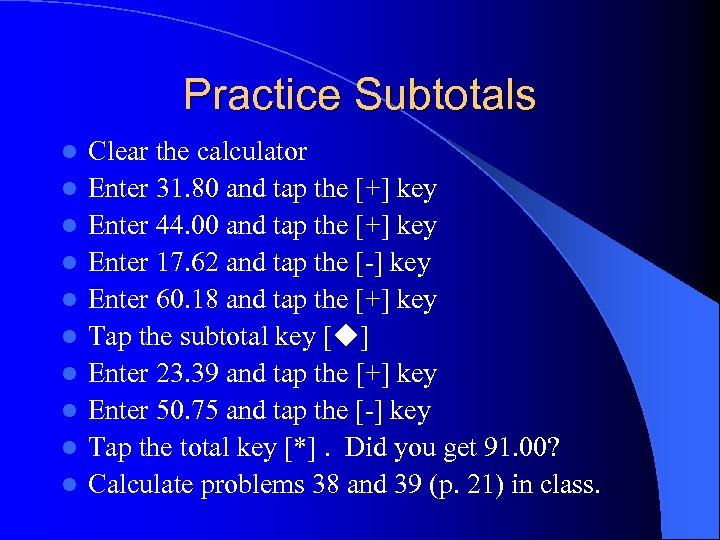Practice Subtotals l l l l l Clear the calculator Enter 31. 80 and tap the [+] key Enter 44. 00 and tap the [+] key Enter 17. 62 and tap the [-] key Enter 60. 18 and tap the [+] key Tap the subtotal key [ ] Enter 23. 39 and tap the [+] key Enter 50. 75 and tap the [-] key Tap the total key [*]. Did you get 91. 00? Calculate problems 38 and 39 (p. 21) in class.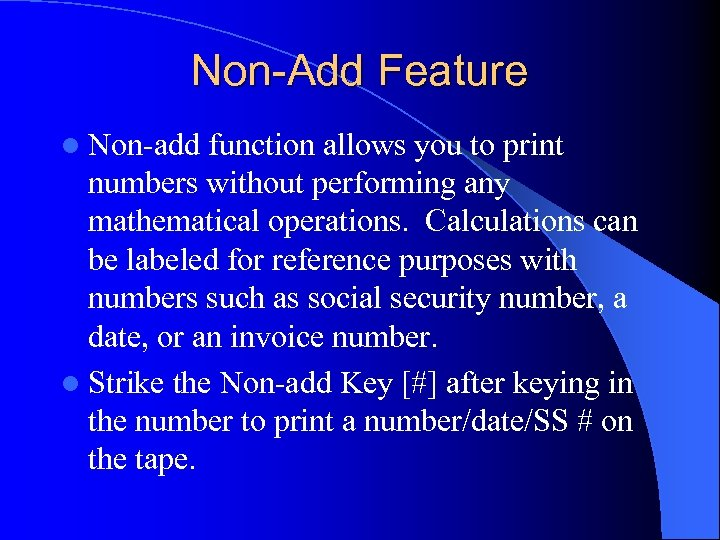Non-Add Feature l Non-add function allows you to print numbers without performing any mathematical operations. Calculations can be labeled for reference purposes with numbers such as social security number, a date, or an invoice number. l Strike the Non-add Key [#] after keying in the number to print a number/date/SS # on the tape.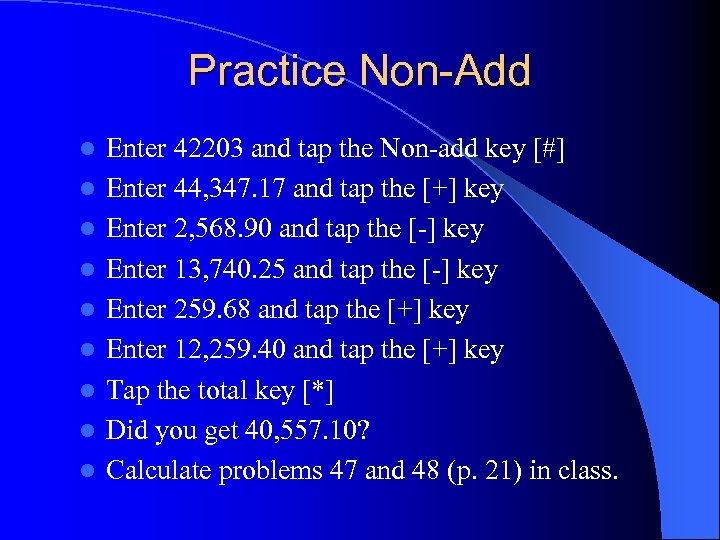Practice Non-Add l l l l l Enter 42203 and tap the Non-add key [#] Enter 44, 347. 17 and tap the [+] key Enter 2, 568. 90 and tap the [-] key Enter 13, 740. 25 and tap the [-] key Enter 259. 68 and tap the [+] key Enter 12, 259. 40 and tap the [+] key Tap the total key [*] Did you get 40, 557. 10? Calculate problems 47 and 48 (p. 21) in class.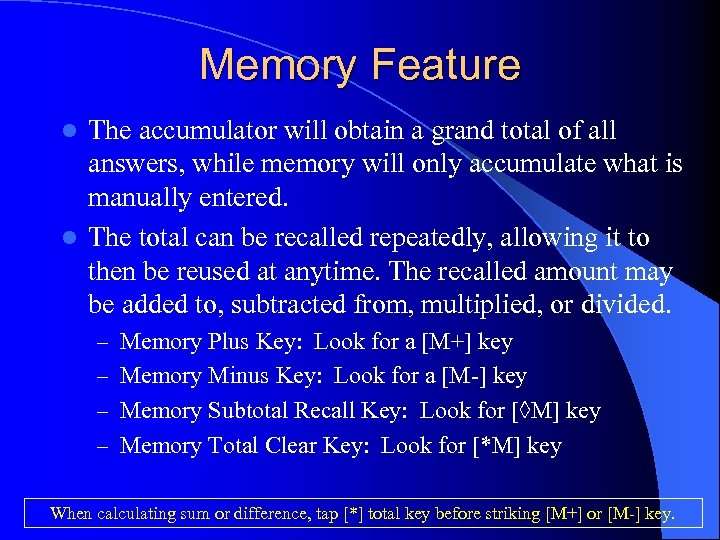Memory Feature The accumulator will obtain a grand total of all answers, while memory will only accumulate what is manually entered. l The total can be recalled repeatedly, allowing it to then be reused at anytime. The recalled amount may be added to, subtracted from, multiplied, or divided. l – Memory Plus Key: Look for a [M+] key – Memory Minus Key: Look for a [M-] key – Memory Subtotal Recall Key: Look for [◊M] key – Memory Total Clear Key: Look for [*M] key When calculating sum or difference, tap [*] total key before striking [M+] or [M-] key.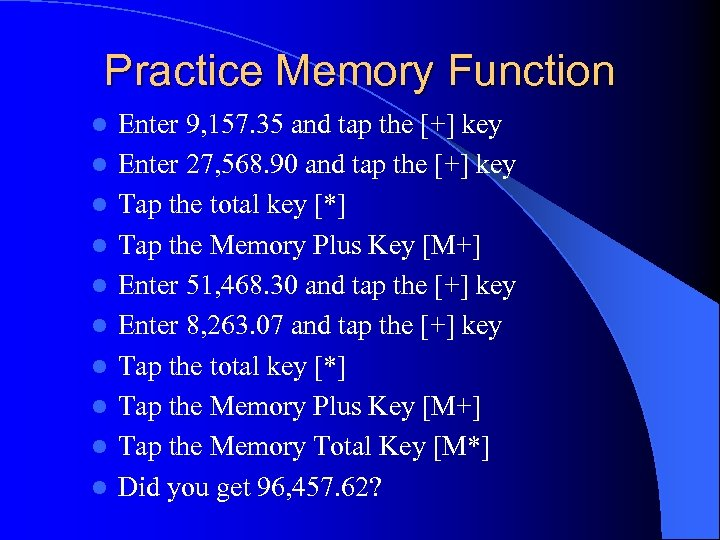Practice Memory Function l l l l l Enter 9, 157. 35 and tap the [+] key Enter 27, 568. 90 and tap the [+] key Tap the total key [*] Tap the Memory Plus Key [M+] Enter 51, 468. 30 and tap the [+] key Enter 8, 263. 07 and tap the [+] key Tap the total key [*] Tap the Memory Plus Key [M+] Tap the Memory Total Key [M*] Did you get 96, 457. 62?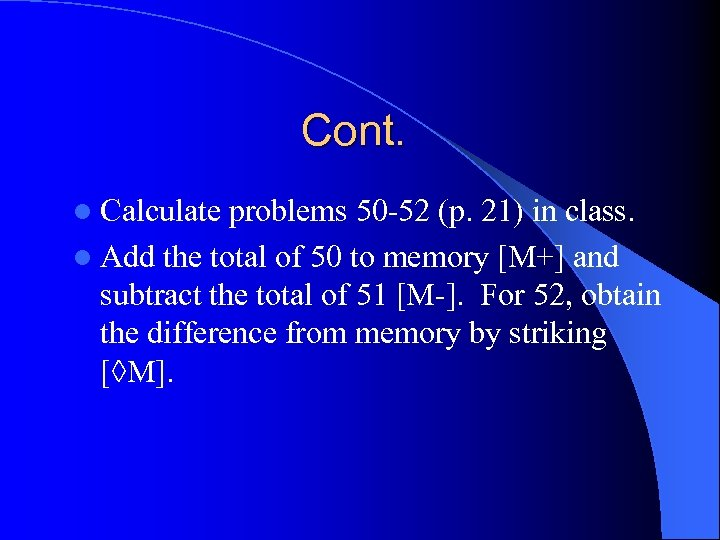Cont. l Calculate problems 50 -52 (p. 21) in class. l Add the total of 50 to memory [M+] and subtract the total of 51 [M-]. For 52, obtain the difference from memory by striking [◊M].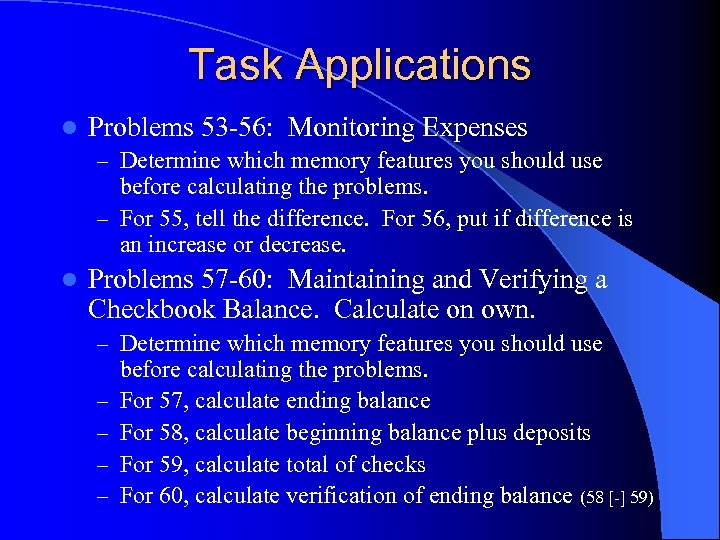Task Applications l Problems 53 -56: Monitoring Expenses – Determine which memory features you should use before calculating the problems. – For 55, tell the difference. For 56, put if difference is an increase or decrease. l Problems 57 -60: Maintaining and Verifying a Checkbook Balance. Calculate on own. – Determine which memory features you should use – – before calculating the problems. For 57, calculate ending balance For 58, calculate beginning balance plus deposits For 59, calculate total of checks For 60, calculate verification of ending balance (58 [-] 59)